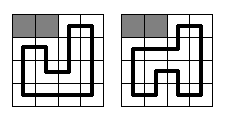# ural 1519（插头DP入门题）

## 1519. Formula 1

Time Limit: 1.0 second
Memory Limit: 16 MB

### Background

Regardless of the fact, that Vologda could not get rights to hold the Winter Olympic games of 20**, it is well-known, that the city will conduct one of the Formula 1 events. Surely, for such an important thing a new race circuit should be built as well as hotels, restaurants, international airport - everything for Formula 1 fans, who will flood the city soon. But when all the hotels and a half of the restaurants were built, it appeared, that at the site for the future circuit a lot of gophers lived in their holes. Since we like animals very much, ecologists will never allow to build the race circuit over the holes. So now the mayor is sitting sadly in his office and looking at the map of the circuit with all the holes plotted on it.

### Problem

Who will be smart enough to draw a plan of the circuit and keep the city from inevitable disgrace? Of course, only true professionals - battle-hardened programmers from the first team of local technical university!.. But our heroes were not looking for easy life and set much more difficult problem: "Certainly, our mayor will be glad, if we find how many ways of building the circuit are there!" - they said.
It should be said, that the circuit in Vologda is going to be rather simple. It will be a rectangleN*M cells in size with a single circuit segment built through each cell. Each segment should be parallel to one of rectangle's sides, so only right-angled bends may be on the circuit. At the picture below two samples are given for N = M = 4 (gray squares mean gopher holes, and the bold black line means the race circuit). There are no other ways to build the circuit here.### Input

The first line contains the integer numbers N and M (2 ≤ NM ≤ 12). Each of the next N lines contains M characters, which are the corresponding cells of the rectangle. Character "." (full stop) means a cell, where a segment of the race circuit should be built, and character "*" (asterisk) - a cell, where a gopher hole is located.

### Output

You should output the desired number of ways. It is guaranteed, that it does not exceed 263-1.

### Samples

input output
4 4
**..
....
....
....

2

4 4
....
....
....
....

6

Problem Author: Nikita Rybak, Ilya Grebnov, Dmitry Kovalioff
Problem Source: Timus Top Coders: Third Challenge

2012-9-27

重新写了下：

#include<cstdio>
#include<cstring>
using namespace std;
const int mm=15511;
typedef long long LL;
struct hashTable
{
int h[mm],s[mm],p[mm],t;
LL v[mm];
void insert(int w,LL val)
{
int i,id=w%mm;
for(i=h[id];i>=0;i=p[i])
if(s[i]==w)
{
v[i]+=val;
return;
}
v[t]=val,s[t]=w,p[t]=h[id],h[id]=t++;
}
void clear()
{
t=0,memset(h,-1,sizeof(h));
}
}f;
bool g;
int i,j,k,n,m,em,g1,g2;
bool ok(int s)
{
if(s==1)return g[i+1][j];
if(s==2)return g[i][j+1];
if(s==3)return g[i+1][j]&&g[i][j+1];
}
{
int n=1,w,x=3<<(j<<1),a=(flag?1:2)<<(j<<1);
while(n)
{
if(flag)a<<=2,x<<=2;
else a>>=2,x>>=2;
w=s&x;
if(w)n+=(w==a)?1:-1;
}
return s^x;
}
void Work(int s,LL val)
{
int e,w=j<<1,ss=(s>>w)&15;
if(ss==9)return;
if(!ss)
{
if(ok(3))f[g2].insert(s|(9<<w),val);
}
else if(!(ss&3)||!(ss&12))
{
if(ss&3)e=1,ss|=ss<<2;
else e=0,ss|=ss>>2;
if(ok(1+!e))f[g2].insert(s,val);
if(ok(1+e))f[g2].insert(s^(ss<<w),val);
}
else if(ss==6)f[g2].insert(s^(ss<<w),val);
}
void end()
{
while(n--)
for(em=m-1;em>=0;--em)
if(g[n][em])return;
}
LL PlugDP()
{
end();
f.clear();
f.insert(0,1);
for(g2=i=0;i<=n;++i)
{
for(k=0;k<f[g2].t;++k)f[g2].s[k]<<=2;
for(j=0;j<m;++j)
if(g[i][j])for(g1=g2,g2=!g2,f[g2].clear(),k=0;k<f[g1].t;++k)
{
if(i==n&&j==em)
if(((f[g1].s[k]>>(j<<1))&15)==9)return f[g1].v[k];
else continue;
Work(f[g1].s[k],f[g1].v[k]);
}
}
return 0;
}
char c;
int main()
{
scanf("%d%d",&n,&m);
memset(g,0,sizeof(g));
for(i=0;i<n;++i)
for(j=0;j<m;++j)
scanf(" %c",&c),g[i][j]=(c=='.');
printf("%I64d\n",PlugDP());
return 0;
}


©️2019 CSDN 皮肤主题: 大白 设计师: CSDN官方博客